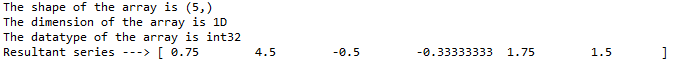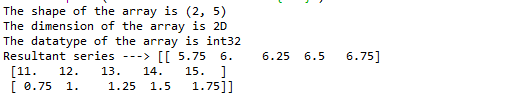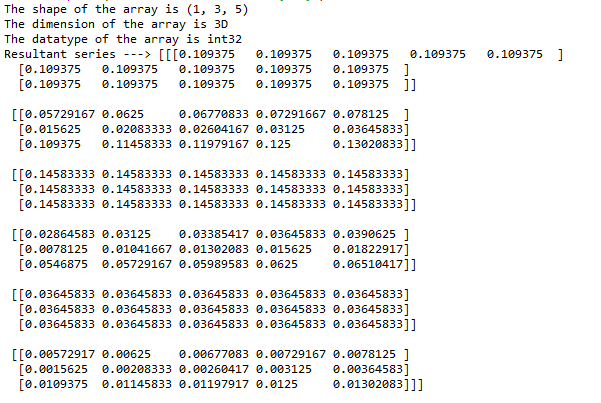GeeksforGeeks App
Open AppBrowser
Continue

# Python Program to Integrate a Chebyshev Series and Set the Integration Constant

In this article, we will discuss how to integrate a Chebyshev Series and set the integration constant

To perform Chebyshev integration, NumPy provides a function called chebyshev.chebint which can be used to integrate Chebyshev series.

Syntax: chebyshev.chebint(c, m=1, k=[], lbnd=0, scl=1, axis=0)

Parameters:

• c – Array of Chebyshev series coefficients.
• m – (integer) Order of integration, must be positive
• k –  Integration constant. The value of the first integral at zero is the first value in the list, the value of the second integral at zero is the second value, etc
• lbnd – The lower bound of the integral. (Default: 0)
• scl – Following each integration the result is multiplied by scl before the integration constant is added. (Default: 1)
• axis – Axis over which the integral is taken.

### Example 1:

In the first example. let us consider a 1D array with a first-order integration and 3 as an integration constant. Import the necessary packages as shown and pass the appropriate parameters as shown below.

## Python3

 `import` `numpy as np``from` `numpy.polynomial ``import` `chebyshev`` ` `# co.efficient array``c ``=` `np.array([``11``, ``12``, ``13``, ``14``, ``15``])`` ` `print``(f``'The shape of the array is {c.shape}'``)``print``(f``'The dimension of the array is {c.ndim}D'``)``print``(f``'The datatype of the array is {c.dtype}'``)`` ` `res ``=` `chebyshev.chebint(c, m``=``1``, k``=``3``)`` ` `# integrated chebyshev series ``# with integration constant of 1``print``(f``'Resultant series ---> {res}'``)`

Output:### Example 2:

In the second example. let us consider a 2D array with a first-order integration and 5 as an integration constant. Import the necessary packages as shown and pass the appropriate parameters as shown below.

## Python3

 `import` `numpy as np``from` `numpy.polynomial ``import` `chebyshev`` ` `# co.efficient array``c ``=` `np.array([[``11``, ``12``, ``13``, ``14``, ``15``], [``3``, ``4``, ``5``, ``6``, ``7``]])`` ` `print``(f``'The shape of the array is {c.shape}'``)``print``(f``'The dimension of the array is {c.ndim}D'``)``print``(f``'The datatype of the array is {c.dtype}'``)`` ` `res ``=` `chebyshev.chebint(c, m``=``1``, k``=``5``)`` ` `# integrated chebyshev series``# with integration constant of 5``print``(f``'Resultant series ---> {res}'``)`

Output:### Example 3:

In the third example. let us consider a 3D array with a fifth-order integration and 7 as an integration constant. Import the necessary packages as shown and pass the appropriate parameters as shown below.

## Python3

 `import` `numpy as np``from` `numpy.polynomial ``import` `chebyshev`` ` `# co.efficient array``c ``=` `np.array([[[``11``, ``12``, ``13``, ``14``, ``15``],``               ``[``3``, ``4``, ``5``, ``6``, ``7``],``               ``[``21``, ``22``, ``23``, ``24``, ``25``]]])`` ` `print``(f``'The shape of the array is {c.shape}'``)``print``(f``'The dimension of the array is {c.ndim}D'``)``print``(f``'The datatype of the array is {c.dtype}'``)`` ` `res ``=` `chebyshev.chebint(c, m``=``5``, k``=``7``)`` ` `# integrated chebyshev series``# with integration constant of 7``print``(f``'Resultant series ---> {res}'``)`

Output:My Personal Notes arrow_drop_up